Smartick is a fun way to learn math!Dec29

# Multiplication Tables: How to Work Them out Using a Grid

We’ve already spent a lot of time talking about multiplication tables on the Smartick Blog. In previous posts, we’ve seen different ways of learning them. Take a look at the links below if you want to see any of them:

## How to make multiplication tables with the help of a grid

Now we’re going to take a look at how to make a multiplication tables grid. The first thing we’re going to do is create a 10×10 grid (if you want to make a multiplication table for numbers larger than 10, you’ll have to make a bigger table).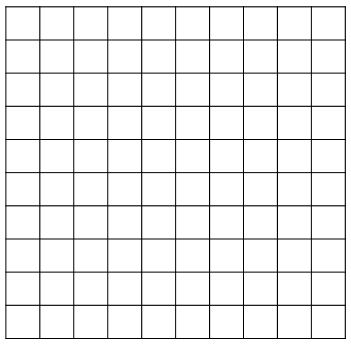We put the zero in the bottom left corner, like so. This is the point that we start to count from.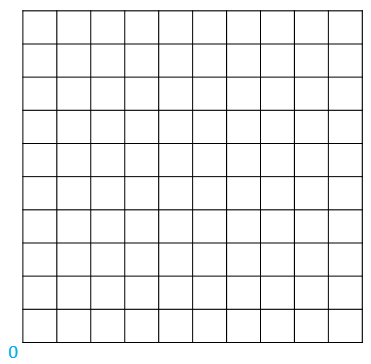Let’s look at an example: 3 x 4

To solve the multiplication, we need to count vertically the same number of squares as the first number that we’re multiplying. In this case, we’re going to count squares.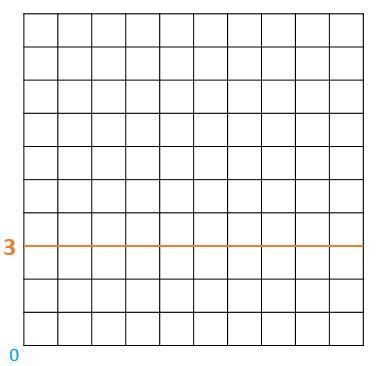Now we do the same with the second number that we’re multiplying, but this time we count horizontally. Once we’ve counted 4 square horizontally, we get something like this: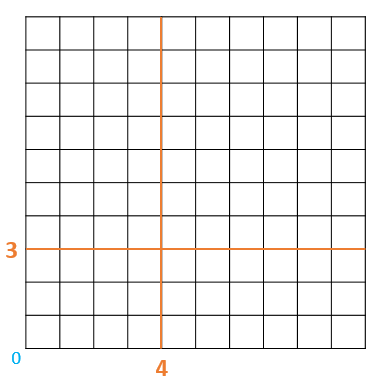We color in the area outlined by the two numbers. To work out the result of the multiplication, we need to count the number of colored squares.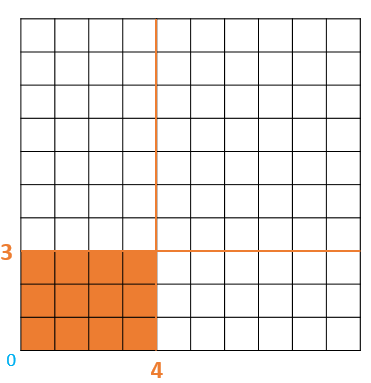The result is 12 squares. Therefore, 3 x 4 = 12

If you’ve found this method of working out times tables interesting, log in to Smartick to find more manipulative material and try it for free.